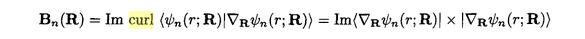# Berry phase, Bra-Ket and gradient

• I
Could somebody show me how to derive this equation? How can I get right side from left. Step by step, thanks....#### Attachments

vanhees71
Gold Member
I've no clue, what your notation means. We need more context!

I only want to know how to solve this curl in left side when I have gradient and ket-bra vectors. I suppose it is elementary vector algebra. It’s simply a math. I need to show that solution of this curl is right side of this equation in this form.

R (external variables) is set of parameters (R1, R2, R3…). In this section, for sake of simplicity, the parameter space R is assumed to span an ordinary three dimensional space. We don’t need generalization of vector algebra to a multi-dimensional space. ∂/∂R=∇R. We consider eigenfunctions psi. Internal variables of the system are collectively indicated by r.

vanhees71
Gold Member
Then it's even more puzzling. What should a cross product between a ket and a bra mean? Again, we need the context and a clear definition of your notation!

PeterDonis
Mentor
2020 Award
this equation?

Where does this equation come from?